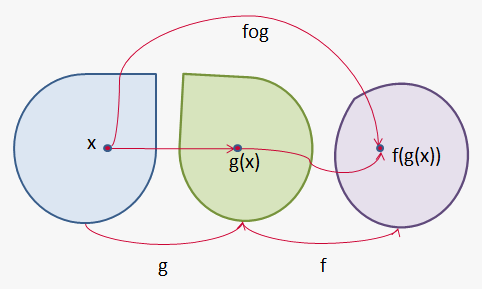# Composition of Functions

Suppose that y=f(u)=ln(u) and u=g(x)=sin(x) . Since y is a function of u and u is afunction of x the we obtain that y is a function of x: y=f(u)=f(g(x))=f(sin(x))=ln(sin(x)) .

The procedure is called composition because the new function is composed of the two given functions f and g.If we are given two functions f and g, we start with a number x in the domain of g and find value g(x). If this number is in the domain of f, then we can calculate the value of f(g(x)). The result is a new function h(x)=f(g(x)) obtained by substituting g into f.

Definition. Given two functions f and g, the composite function f@g is defined by (f@g)(x)=f(g(x)).

Domain of composite functions is set of all x such that x is in domain of g and g(x) is in domain of f.

Example 1. Find f@g, g@f, f@f, g@g if f(x)=x^3 and g(x)=sqrt(x)/(x-1) .

f@g=f(g(x))=f(sqrt(x)/(x-1))=(sqrt(x)/(x-1))^3

g@f=g(f(x))=g(x^3)=sqrt(x^3)/(x^3-1) .

Notice, that in general f@g!=g@f .

f@f=f(f(x))=f(x^3)=(x^3)^3=x^9

g@g=g(g(x))=g(sqrt(x)/(x-1))=sqrt(sqrt(x)/(x-1))/(sqrt(x)/(x-1)-1).

Note, that we can take composition of more than two functions: (f@g@h)(x)=f(g(h(x))).

Example 2. Find f@g@h if f(x)=x^2, g(x)=x/(x-1) and h(x)=sin(x).

f@g@h=f(g(h(x)))=f(g(sin(x)))=f(sin(x)/(sin(x)-1))=(sin(x)/(sin(x)-1))^2.

Sometimes we need to perform inverse task, in other words given composite function, we need to find functions it is formed from.

Example 3. Find f, g, and h if f@g@h=(cos(x+9))^2.

The formula for f@g@h says: first add 9, then take the cosine of the result, and finally square.

So, we take h(x)=x+9, g(x)=cos(x) and f(x)=x^2.

Then

f@g@h=f(g(h(x)))=f(g(x+9))=f(cos(x+9))=(cos(x+9))^2.

It is worth noting, that characteristic of function as composite is not connected with natural functional dependence of f and x, it is just a way to represent this dependence.

For example, let f=sqrt(1-u^2) for u in[-1,1] and y=sin(x) for x in [-pi/2,pi/2] then (f@g)(x)=f(g(x))=f(sin(x))=sqrt(1-(sin(x))^2)=cos(x). Here cos(x) is represented as composite function.